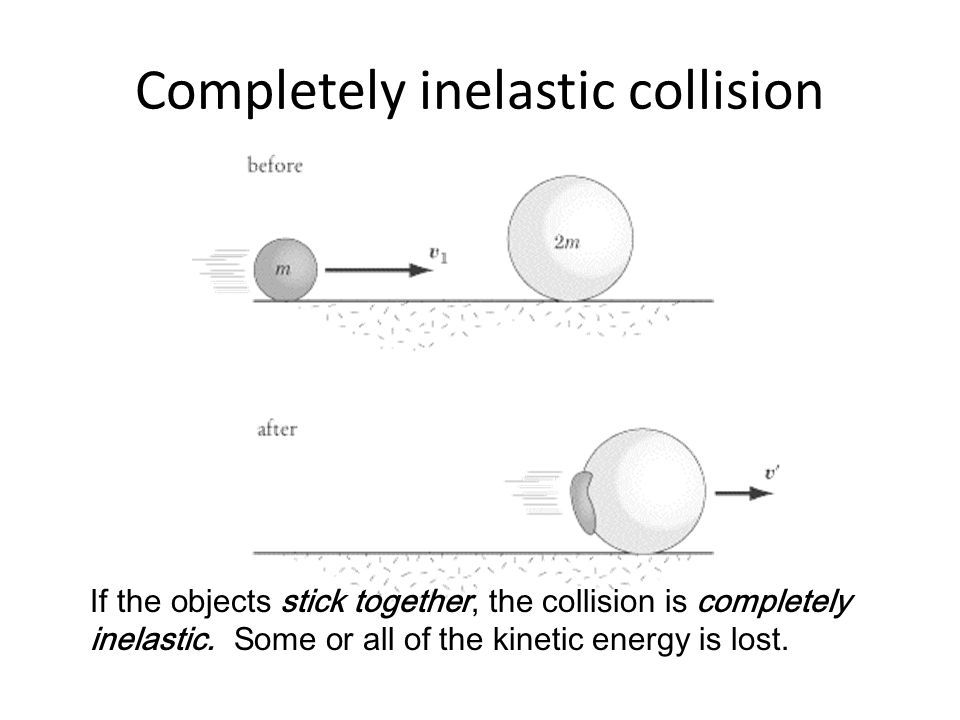top of page
Search

# Collisions

There are different types of collisions in physics. First let's define collision. What is collision exactly? Collision is an instance of one moving object or person striking violently against another. Collision is an event where momentum or kinetic energy is transferred from one object to another. Momentum (p) is the product of mass and velocity (p = mv).

Different types of collisions are:

1. Elastic collision

In elastic collision the total kinetic energy of the objects does not change after the collision or a better way to say it is that both momentum and kinetic energy are conserved.

KE=KE'

P=P'2. Inelastic Collision

In this type of collision the total kinetic of the objects after the collisions is different from the total kinetic energy of them before the collision. Inelastic collision occurs when two objects collide and kinetic energy is not conserved. In this type of collision some of the initial kinetic energy is converted into other types of energy (heat, sound, etc.), which is why kinetic energy is NOT conserved in an inelastic collision.

KE <> KE'

P = P'

3. Completely Inelastic Collision

In a perfectly inelastic collision, the two objects colliding stick together and therefore, have the same velocity. Kinetic energy after the collision in this type, experiences a maximum decrease.

KE' < KE

P = P'In reality, most collisions fall somewhere between the extremes of a completely elastic collision and a completely inelastic collision.

To understand the whole concept, try solving this problem and watching the following videos.

Question: Two billiard balls collide. Ball 1 moves with a velocity of 4 m/s, and ball 2 is at rest. After the collision, ball 1 comes to a complete stop. What is the velocity of ball 2 after the collision? Is this collision elastic or inelastic? The mass of each ball is 0.16 kg.

In the video below, we can see some examples of collisions in real life.